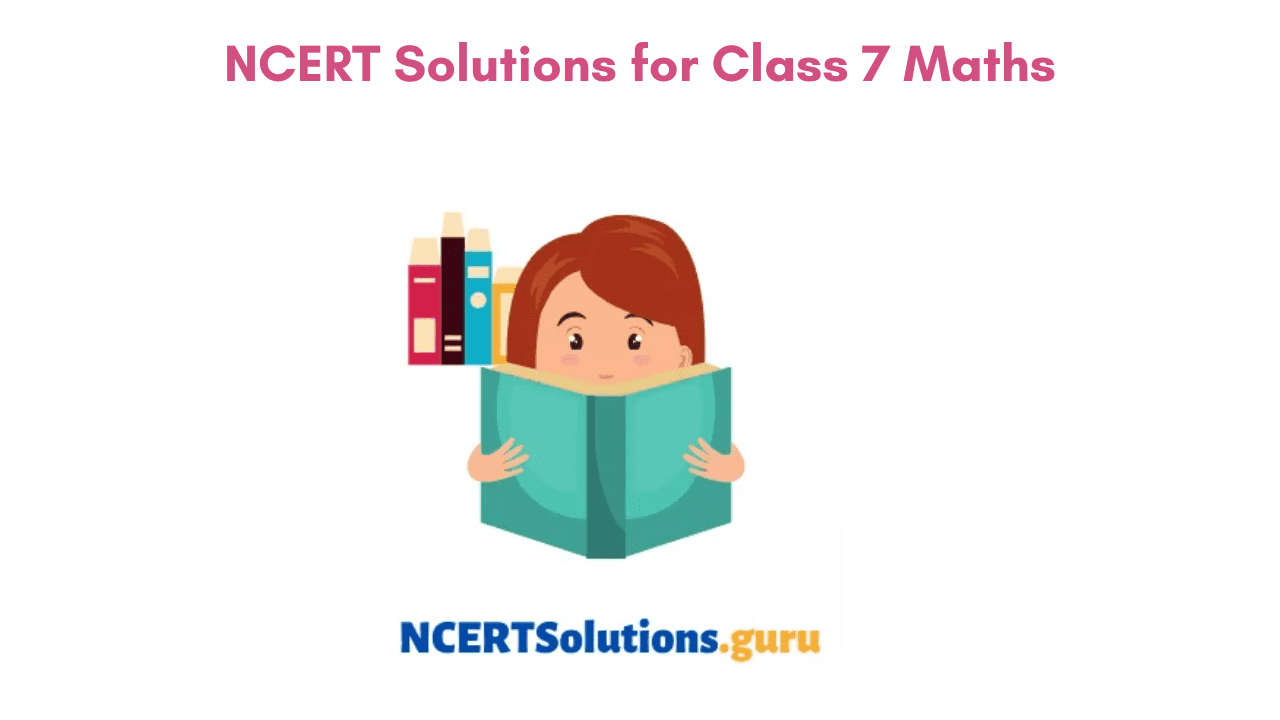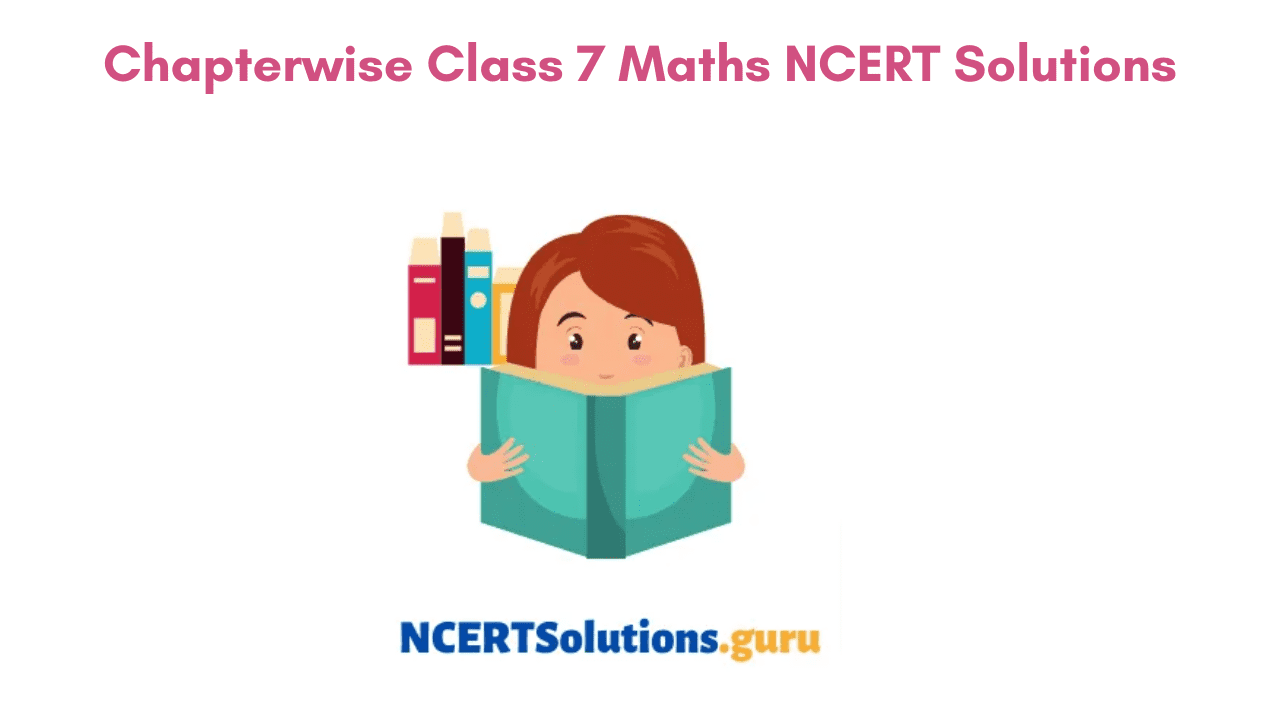# NCERT Solutions for Class 7 Maths | CBSE Class 7th Maths Solutions for All Chapters

An Ultimate need for students for practicing math concepts is NCERT Solutions of Class 7 Maths. One can refer to these 7th Class Maths NCERT Solutions if they have any trouble in solving the questions from NCERT Textbooks. NCERT Solutions for Class 7 Maths are part of NCERT Solutions for Class 7. Class 7 Maths NCERT Solutions are prepared by highly qualified teachers and have prepared all the solutions in a simple and easy-to-understand language.

Class 7th NCERT Solutions for Maths provided on our page will assist you to understand the concepts from a deeper level. Students of Class 7 can use the PDF links to access the Chapter Wise NCERT Solutions of 7th Class Maths and prepare the corresponding topics within. By answering the Questions from Class 7 NCERT Maths Solutions you can get an idea of how to frame better answers and thereby achieve better academic scores.

## Chapterwise Class 7 Maths NCERT Solutions | NCERT Solutions of Class 7 Maths

Students are advised to refer through the Class 7th Maths NCERT Solutions for All Chapters aligned as per the CBSE(https://www.cbse.gov.in/) Guidelines. All the Questions provided are explained in a comprehensive manner so that it’s easy for you to understand the logic behind each and every problem. Use the NCERT Chapterwise Solutions for Class 7 Maths in Hindi and English Medium Updated for Academic Year 2021-22. Improve your math calculations at a faster note by downloading the Maths NCERT Solutions of Grade 7 for free.### Class 7 Maths Chapter 1 Integers

In the earlier classes, you might have studied about whole numbers, integers concepts. This Chapter gives you much in detail about Integers, Properties and Operations performed on them. Make use of the Exercisewise NCERT Solutions of Chapter 1 by simply accessing the quick links available.

### Class 7 Maths Chapter 2 Fractions and Decimals

Chapter 2 of Class 7 NCERT Books deals with fractions and decimals in major. You will learn about different operations performed on Fractions and Decimals like Addition, Subtraction, Multiplication, and Division. In Multiplication of Fractions and Decimals, you will come across various topics such as Multiplication of a Fraction by Whole Number, Multiplication of Fraction by Fraction, Dividing the Whole Number by Fraction, Multiplying Decimal by a Decimal, Multiplying Decimal with the Whole Number, etc.

Just click on the exercises links to prepare the problems within the above-listed topics in no time.

### Class 7 Maths Chapter 3 Data Handling

In this Chapter 3 data handling, you will learn various types of data. Know how to collect data and frame it in terms of bar graphs. Get topics about collecting data, organizing data, representative value, mean and median of large data, probability, etc. Practice the Ex 3.1, 3.2, 3.3, 3.4 NCERT Solutions of Class 7 Maths through the direct links available below.

### Class 7 Maths Chapter 4 Simple Equations

Chapter 4 for Simple Equations gives you an idea of solving simple equations that would include moving from one side to another, finding the transpose of a number. Learn applications of simple equations in practical scenarios. Exercise Solutions for all the topics covered in Chapter 4 Class 7 Maths are explained below.

### Class 7 Maths Chapter 5 Lines and Angles

Chapter 5 Lines and Angles have topics covering related angles, supplementary angles, complementary angles,  adjacent angles, vertically opposite angles, linear pairs, pairs of lines intersecting lines, transversal, a transversal of parallel lines, the angle made by a transversal, checking of parallel lines.

### Class 7 Maths Chapter 6 The Triangles and Its Properties

Triangle and Its Properties has concepts on finding median of a triangle, altitude of a triangle, angle sum property of a triangle, exterior angle of a triangle, and its property, sum of the lengths of two sides of a triangle, right-angled triangles, and Pythagoras property.

### Class 7 Maths Chapter 7 Congruence of Triangles

Congruence of Triangles Chapter covers concepts for congruence of plane figures, congruence of angles, congruence of triangles, congruence among line segments, congruence of right-angled triangles, criteria for congruence of triangles. Make use of Exercise Wise Solutions for Chapter 7 Class 7 Maths via quick links available below.

### Class 7 Maths Chapter 8 Comparing Quantities

In the Comparing Quantities Chapter 7, you will find topics for finding equivalent ratios, the meaning of percentage, converting decimals to percentage, converting fractions to percentage. Also, learn other topics like increase or decrease percent, profit or loss as a percentage, etc.

### Class 7 Maths Chapter 9 Rational Numbers

NCERT Textbook for Class 7 Ch 9 deals with Rational Numbers like expressing rational numbers on a number line, finding positive and negative rational numbers, operations on rational numbers, comparing rational numbers, etc.

### Class 7 Maths Chapter 10 Practical Geometry

In Chapter 10 Practical Geometry you will come across different shapes and how to draw them. Get to know the topics like how to draw a line parallel to a given line, angle bisector, a line perpendicular to a given line segment,  constructing different types of triangles, etc.

### Class 7 Maths Chapter 11 Perimeter and Area

In the earlier classes, you might have learned about finding the area and perimeter of plane figures like squares, rectangles. In this chapter, you will learn to find the area and perimeter for few other figures in detail. Avail the Exercise Wise Solutions for (11.1-11.4) using the quick links listed below.

### Class 7 Maths Chapter 12 Algebraic Expressions

NCERT Textbook for Class 7 Ch 12 deals with terms coefficients, expression, like and unlike terms, monomials, binomials, finding values of an expression.

### Class 7 Maths Chapter 13 Exponents and Powers

Chapter 13 Exponents and Powers deals with the topic Exponents and Powers. Concepts explained in the chapter are laws of exponents, multiplying powers having the same bases, finding the power of a power, multiplying powers with the same exponents, expressing large numbers in standard form, etc. Use the NCERT Solutions for Class 7 Maths Ch 7 to aid our preparation and understand the concepts.

### Class 7 Maths Chapter 14 Symmetry

Symmetry is all about making two figures look like one another by moving upside down, turning, or by flipping. In Chapter 14 for Symmetry, you will come across rotational symmetry, line symmetry of regular polygons, etc.

### Class 7 Maths Chapter 15 Visualising Solid Shapes

NCERT Textbook covering Chapter 15 deals with plane figures and solids, edges, faces, vertices, drawing solids on a flat surface, nets for building 3-D shapes, isometric sketches, oblique sketches, etc.

### Advantages of preparing from Class 7 Maths NCERT Solutions

Go through the simple and easy steps listed below to avail the benefits of studying from NCERT Solutions of Class 7th Maths. They are as follows

• Maths Class 7 NCERT Solutions provided are explained in a simple and comprehensive manner.
• Chapterwise Class 7th Maths NCERT Solutions given in PDF Format makes it easy for students to download and prepare offline.
• You can gain in-depth knowledge on the respective topics since all the solutions are explained in a step-by-step manner.
• Class 7 Maths NCERT Solutions is as per the latest CBSE Syllabus Guidelines.### FAQs on CBSE Chapter Wise NCERT Solutions 7th Class Maths

1. Is there any portal that provides the best study material for Class 7 Maths NCERT Solutions?

NCERTSolutions.guru is a genuine and reliable site that provides the Class 7 Maths NCERT Solutions organized efficiently.

You can download NCERT Solutions for Class 7 Maths by simply tapping on the quick links available.

3. Where do I get Chapter Wise Class 7th Maths NCERT Solutions PDF?

You can get Chapter Wise Class 7th Maths NCERT Solutions PDF for free on our page.

### Summary

Hope the information shared on NCERT Solutions for Class 7 Maths is true and genuine. If you need any suggestions do ask us via the comment section so that we can revert back to you with a possible solution. Bookmark our site for more updates on NCERT Solutions for 7th Grade along with Other Grades.

#### Class 7 Study Material

error: Content is protected !!